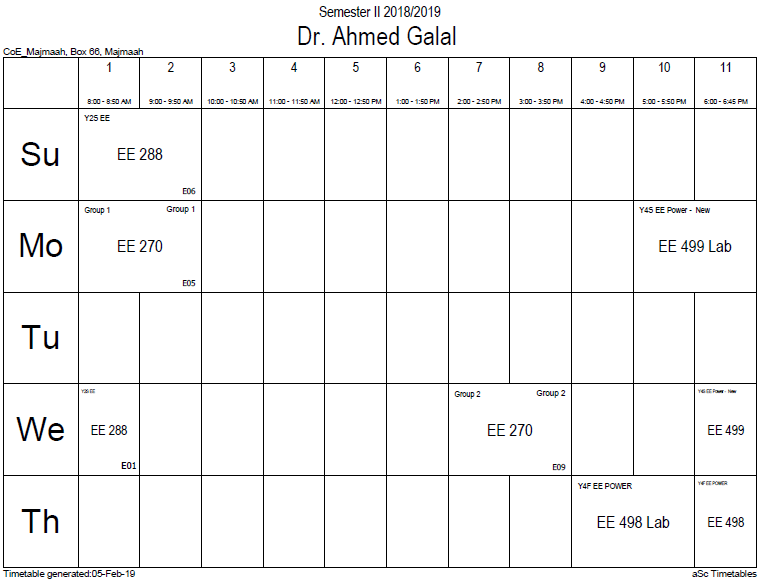## Reluctance

Magnetic reluctance, or magnetic resistance, is analogous to resistance in an electrical circuit (although it does not dissipate magnetic energy). In likeness to the way an electric field causes an electric current to follow the path of least resistance, a magnetic field causes magnetic flux to follow the path of least magnetic reluctance. It is a scalar, extensive quantity, akin to electrical resistance.

The total reluctance is equal to the ratio of the (MMF) in a passive magnetic circuit and the magnetic flux in this circuit. In an AC field, the reluctance is the ratio of the amplitude values for a sinusoidal MMF and magnetic flux. (see phasors)

The definition can be expressed as:$mathcal{R} = frac{mathcal{F}}{Phi},$

where$scriptstyle mathcal{R}$ is the reluctance in ampere-turns per weber (a unit that is equivalent to turns per henry).

Magnetic flux always forms a closed loop, as described by Maxwell's equations, but the path of the loop depends on the reluctance of the surrounding materials. It is concentrated around the path of least reluctance. Air and vacuum have high reluctance, while easily magnetized materials such as soft iron have low reluctance. The concentration of flux in low-reluctance materials forms strong temporary poles and causes mechanical forces that tend to move the materials towards regions of higher flux so it is always an attractive force(pull).

The inverse of reluctance is called permeance.$mathcal{P} = frac{1}{mathcal{R}}$

Its SI derived unit is the henry (the same as the unit of inductance, although the two concepts are distinct).

## Microscopic origins of reluctance

The reluctance of a magnetically uniform magnetic circuit element can be calculated as:$mathcal{R} = frac{l}{mu A}$

where

l is the length of the element in metres$mu = mu_rmu_0$ is the permeability of the material ($mu_r$ is the relative permeability of the material (dimensionless), and$mu_0$ is the permeability of free space)
A is the cross-sectional area of the circuit in square metres
This is similar to the equation for electrical resistance in materials, with permeability being analogous to conductivity; the reciprocal of the permeability is known as magnetic reluctivity and is analogous to resistivity. Longer, thinner geometries with low permeabilities lead to higher reluctance. Low reluctance, like low resistance in electric circuits, is generally preferred.

### Office HoursNo office hours### My Timetable### Contactsemail: [email protected]

Phone: 2570

### Welcome

Welcome To Faculty of Engineering### IEEEhttp://www.ieee.org/

/

### Bookmarkshttp://www.utk.edu/research/

http://science.doe.gov/grants/index.asp

http://www1.eere.energy.gov/vehiclesandfuels/

http://www.eere.energy.gov/

### Upcoming Conferences### Engineering quotes### Travel Web Siteshttp://www.hotels.com/

http://www.orbitz.com/

http://www.hotwire.com/us/index.jsp

http://www.kayak.com/

### Blackboardستقام اختبارات الميدتيرم يوم الثلاثاء 26-6-1440

حسب الجدول المعلن بلوحات الاعلان

### Summer trainingThe registration for summer training will start from 5th week of second semesterClass registration week 1

### برنامج التجسير### إحصائية الموقع

عدد الصفحات: 2879

البحوث والمحاضرات: 1280

الزيارات: 100940